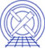AHELP for CIAO 3.4 ftest Context: sherpa

## Synopsis

Computes significance using the F test.

## Syntax

`sherpa> FTEST <dof_1> <stat_1> <dof_2> <stat_2>`

## Description

The command arguments are:

#### Valid FTEST Command Arguments

Argument Description
<dof_1> Number of degrees of freedom (dof) for the fit of the null hypothesis (the simple model).
<stat_1> Best-fit statistic for the null hypothesis.
<dof_2> Number of degrees of freedom in the fit of the alternative hypothesis (more complex model).
<stat_2> Best-fit statistic for the alternative hypothesis.

The F-test is a model comparison test. Model comparison tests are used to select from two competing models that which best describes a particular dataset.

A model comparison test statistic T is created from the best-fit statistics of each fit; as with all statistics, it is sampled from a probability distribution p(T). The test significance is defined as the integral of p(T) from the observed value of T to infinity. The significance quantifies the probability that one would select the more complex model when in fact the null hypothesis is correct. A standard threshold for selecting the more complex model is significance < 0.05 (the "95% criterion" of statistics).

The F-test may be used if:

• the simpler of the two models is nested within the other, i.e., one can obtain the simpler model by setting the extra parameters of the more complex model to default values, often zero or one;
• those normal distributions are not truncated by parameter space boundaries;
• and the best-fit statistics for each fit individually are sampled from the chi-square distribution.

If these conditions are fulfilled, then the observed F statistic is sampled from the F distribution, whose shape is a function of <dof_1> and <dof_2>. (The tail integral may be computed analytically using an incomplete beta function; see any basic statistics text for details.) If these conditions are not fulfilled, then the F-test significance may not be accurate.

One can create three F statistics out of the best-fit statistics for two model fits; the most powerful F-test, at least for line detection, uses the change in statistic from one fit to the next as <stat_1>, the additional number of parameters in the more complex model (<delta_dof>) for <dof_1>, the best-fit of the more complex model for <stat_2>, and the number of degrees of freedom for the more complex model for <dof_2>.

The F-test significance can also be retrieved using the Sherpa/S-Lang module function get_ftest.

## Example

Compute the F-test significance given two fits:

```sherpa> FTEST 2 20.28 34 33.63
significance = 0.000328079
```

As noted above, for line fits, the first two numbers should be the difference in degrees of freedom and fit statistic, respectively, between the null hypothesis (continuum) fit (for which the chi-square is 53.91 for 36 dof), and the alternative hypothesis (continuum-plus-line) fit (for which the chi-square is 33.63 for 34 dof). In general, if the significance is smaller than a predefined threshold (e.g., 0.05), then the more complex model is selected (as it is in this case). Otherwise the null hypothesis is selected.

## Bugs

See the Sherpa bug pages online for an up-to-date listing of known bugs.

Hardcopy (PDF): A4 | LetterThe Chandra X-Ray Center (CXC) is operated for NASA by the Smithsonian Astrophysical Observatory. 60 Garden Street, Cambridge, MA 02138 USA.    Email: cxcweb@head.cfa.harvard.edu Smithsonian Institution, Copyright © 1998-2004. All rights reserved.